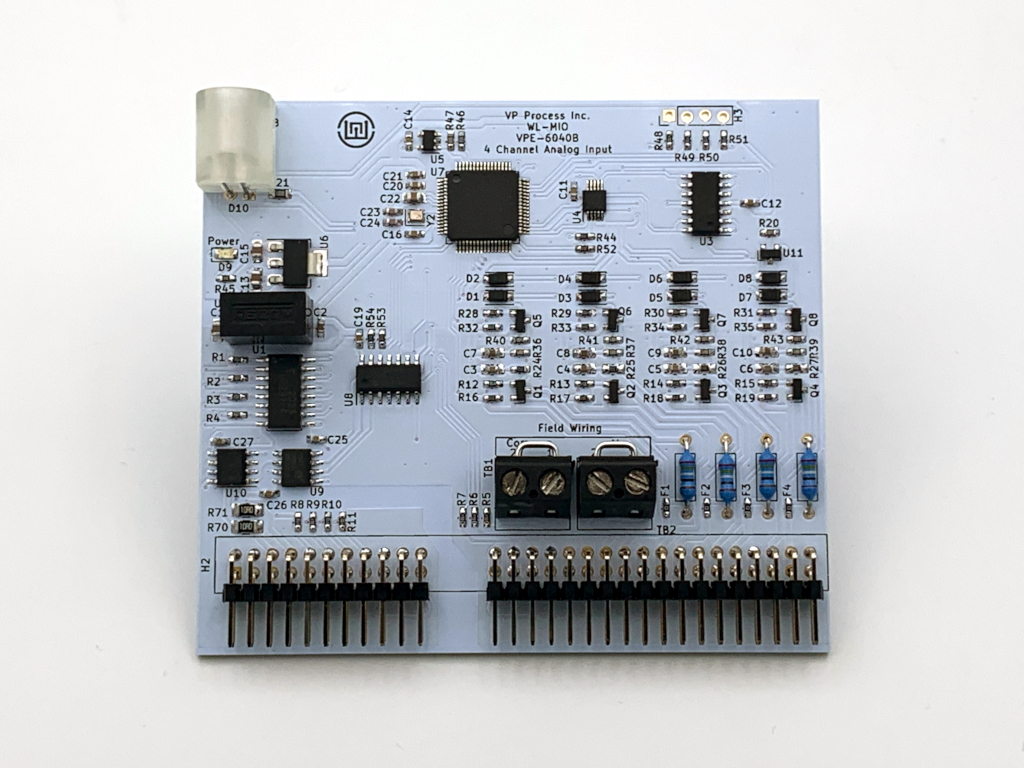### Application Note: WL-MIO-AN-36040

#### 4 Channel Analog Input Module SDAFE (mA and VDC), 15 BitThe VPE-6040 Analog Input 4 Channel SDAFE (Software Defined Analog Front End) Module has 4 analog inputs that can be independantly read and configured. Each input channel can be configured as:

• 0 to 20 mA , Readings are in uA, configure value = 1
• 0 to 5 VDC , Readings are in mV, configure value = 0
• 0 to 10 VDC , Readings are in mV, configure value = 2

The analog input module can be installed in any backplane position. The NodeID referred to in the demo program is the "Backplane ID" determined by the backplane jumpers and the addition of the slot in which the power supply is plugged into.
Example 1: If the backplane has no jumpers and the analog input module is plugged into slot position 0, the NodeID is 0 + 0 = 0.
Example 2: If the backplane has a jumper in the "8" position and the analog input module is plugged into slot position 2, the NodeID is 8 + 2 = 10.

During normal operation, the status LED flashes GREEN once per second as a "heartbeat" indicator that everything is functioning normally. If the onboard firmware detects any fault conditions, the Status LED will flash either AMBER or RED.

For the following demo code to work, the "libwlmio" library has to be installed.

#### Demo Python Code

The Backplane has ID = 0 and the analog input module is plugged into slot postion 2.

```# vpe6040 Analog Input SDAFE 4 Channel Demo Program
# Configure Channels as 0 to 20 mA, 0 to 5 VDC or 0 to 10 VDC
# configure = 0 for 0 to 5 VDC input
# configure = 1 for 0 to 20 mA input
# configure = 2 for 0 to 10 VDC input

import asyncio
from pywlmio import *

NodeID = 2  #NodeID location is the Bacplane ID (Jumpers) and Power Supply Slot location

async def main():
init()

ai = VPE6040(NodeID)

try:
await asyncio.gather(
ai.ch1.configure(1),   # 1 = 0 to 20 mA input
ai.ch2.configure(1),   # 1 = 0 to 20 mA input
ai.ch3.configure(0),   # 0 = 0 to 5 VDC input
ai.ch4.configure(2)    # 2 = 0 to 10 VDC input
)

except WlmioWrongNodeError:
print("Error NodeID = %d Wrong module installed" % NodeID)  # Error Check if wrong type of module installed
except WlmioInternalError:
print("Error NodeID = %d Timed out" % NodeID)               # Error Check - Typically module not installed

while True:
try:
a = await asyncio.gather(
)

print("Module VPE6040 NodeID = %d" % NodeID)
print("Reading Array = ", a)               # Array holds all input channel readings
print("AI 1 = %5.03f mA" % (a/1000))
print("AI 2 = %5.03f mA" % (a/1000))
print("AI 3 = %5.03f VDC" % (a/1000))
print("AI 4 = %5.03f VDC" % (a/1000))
print("")

except WlmioWrongNodeError:
print("Error NodeID = %d Wrong module installed" % NodeID)  # Error Check if wrong type of module installed
except WlmioInternalError:
print("Error NodeID = %d Timed out" % NodeID)               # Error Check - Typically module not installed

await asyncio.sleep(1)

asyncio.run(main(), debug=True)
```

Output from the demo program in a terminal window:

```pi@raspberrypi:~/Desktop/wlmio/wlmio_rpi/src/demos \$ python3 vpe6040_demo.py
Module VPE6040 NodeID = 2
Reading Array =  [4191, 12550, 968, 5662]
AI 1 = 4.191 mA
AI 2 = 12.550 mA
AI 3 = 0.968 VDC
AI 4 = 5.662 VDC
```